# Lecture 12

online, you can click here to see some images created by members of the Electronic Visualization Laboratory here at UIC. Each lecture has a different set of images (collect em all!)

Through the first half of the class polygons were represented by the line segments connecting their vertices, or by filling them with a solid colour. This is not very realistic looking.

Today we are going to talk about how different lighting models are used to make computer graphics look more realistic.

### General Principles

Trying to recreate reality is difficult.

Lighting calculations can take a VERY long time.

The techniques described here are heuristics which produce appropriate results, but they do not work in the same way reality works - because that would take too long to compute, at least for interactive graphics.

Instead of just specifying a single colour for a ploygon we will instead specify the properties of the material that the polygon is supposed to be made out of, and the properties of the light or lights shining onto that material.

### Illumination Models

No Lighting

• There are no lights in the scene
• Each polygon is self-luminous (it lights itself, but does not give off light)
• Each polygon has its own colour which is constant over its surface
• That colour is not affected by anything else in the world
• That colour is not affected by the position or orientation of the polygon in the world
• This is very fast, but not very realistic
• position of viewer is not important

I = Ki
I: intensity
Ki: object's intrinsic intensity, 0.0 - 1.0 for each of R, G, and B

This scene from battalion has no lighting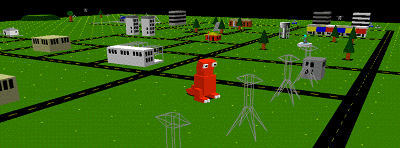Ambient
• Non-directional light source
• Simulates light that has been reflected so many times from so many surfaces it appears to come equally from all directions
• intensity is constant over polygon's surface
• intensity is not affected by anything else in the world
• intensity is not affected by the position or orientation of the polygon in the world
• position of viewer is not important

I = IaKa
I: intensity
Ia: intensity of Ambient light
Ka: object's ambient reflection coefficient, 0.0 - 1.0 for each of R, G, and B

Types of Light Sources
• point light - a light that gives off equal amounts of light in all directions. Polygons, and parts of polygons which are closer to the light appear brighter than those that are further away
• directional light - if a point light is moved to infinity, all of the light rays emanating from the light strike the polygons in the scene from a single direction
• spotlight - light that radiates light in a cone with more light in the center of the cone, gradually tapering off towards the sides of the cone.

Here is the same object (Christina Vasilakis' SoftImage Owl) under different lighting conditions:

bounding boxes of the components of the owl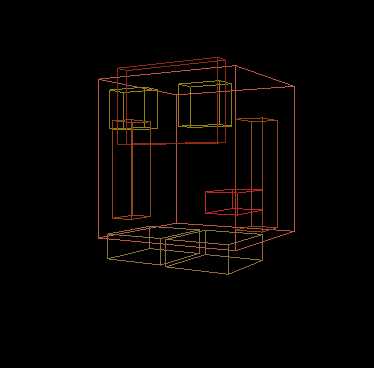self-luminous owl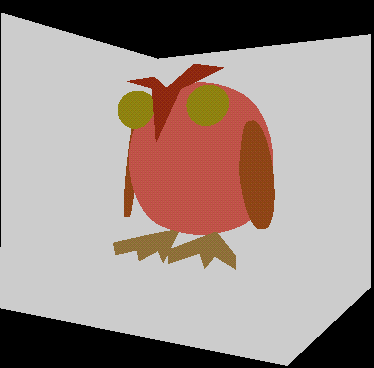directional light from the front of the owl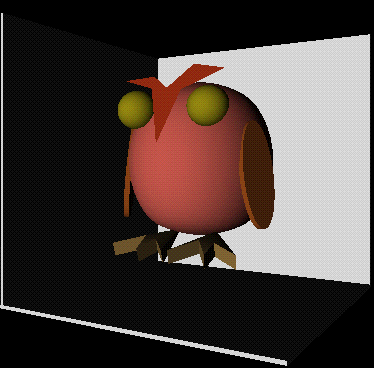point light slightly in front of the owl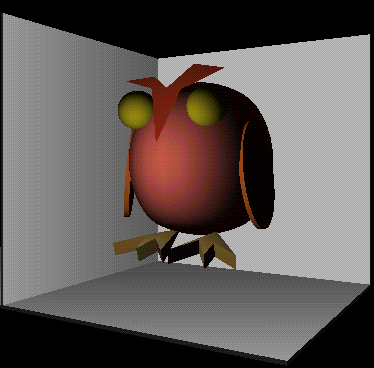spotlight slightly in front of the owl aimed at the owl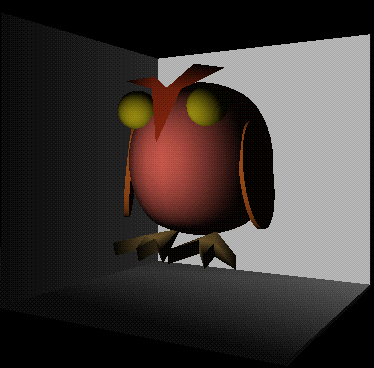Diffuse Reflection (Lambertian Reflection)

Using a point light:

• comes from a specific direction
• reflects off of dull surfaces
• light reflected with equal intensity in all directions
• brightness depends on theta - angle between surface normal (N) and the direction to the light source (L)
• position of viewer is not important

I = Ip Kd cos(theta) or I = Ip Kd(N' * L')
I: intensity
Ip: intensity of point light
Kd: object's diffuse reflection reflection coefficient, 0.0 - 1.0 for each of R, G, and B
N': normalized surface normal
L': normalized direction to light source

Using a directional light:

• theta is constant
• L' is constant

Directional lights are faster than point lights because L' does not need to be recomputed for each polygon.

It is rare that we have an object in the real world illuminated only by a single light. Even on a dark night there is a some ambient light. To make sure all sides of an object get at least a little light we add some ambient light to the point or directional light:

I = Ia Ka + Ip Kd(N' * L')

Currently there is no distinction made between an object close to a point light and an object far away from that light. Only the angle has been used so far. It helps to introduce a term based on distance from the light. So we add in a light source attenuation factor: Fatt.

I = Ia Ka + Fatt Ip Kd(N' * L')

Coming up with an appropriate value for Fatt is rather tricky.

It can take a fair amount of time to balance all the various types of lights in a scene to give the desired effect (just as it takes a fair amount of time in real life to set up proper lighting)

Specular Reflection
• reflection off of shiny surfaces - you see a highlight
• shiny metal or plastic has high specular component
• chalk or carpet has very low specular component
• position of the viewer IS important in specular reflection

I = Ip cosn(a) W(theta)
I: intensity
Ip: intensity of point light
n: specular-reflection exponent (higher is sharper falloff)
W: gives specular component of non-specular materials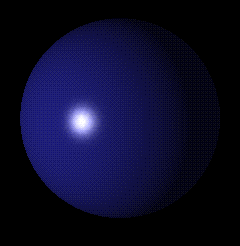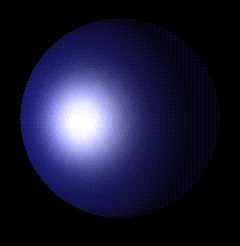So if we put all the lighting models depending on light together we add up their various components to get:

I = Ia Ka + Ip Kd(N' * L') + Ip cosn(a) W(theta)

In OpenGL a polygon can have the following material properties:

• ambientColor (R, G, B)
• diffuseColor (R, G, B)
• specularColor (R, G, B)
• emissiveColor (R, G, B)
• transparency 0.0 - 1.0
• shininess 0.0 - 1.0

These properties describe how light is reflected off the surface of the polygon. a polygon with diffuse color (1, 0, 0) reflects all of the red light it is hit with, and absorbs all of the blue and green. If this red polygon is hit with a white light it will appear red. If it with a blue light, or a green light, or an aqua light it will appear black (as those lights have no red component.) If it is hit with a yellow light or a purple light it will appear red (as the polygon will reflect the red component of the light.)

The following pictures will help to illustrate this:

 ball light image white redred white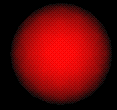red green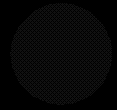purple blue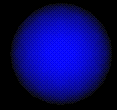yellow aqua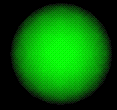`   `

Fog

We talked earlier about how atmospheric affects give us a sense of depth as particles in the air make objects that are further away look less distinct than near objects.

Fog, or atmospheric attenuation allows us to simulate this affect.

Fog is typically given a starting distance, an ending distance, and a colour. The fog begins at the starting distance and all the colours slowly transition to the fog colour towards the ending distance. At the ending distance all colours are the fog colour.

Here are those o-so-everpresent computer graphics teapots from the OpenGL samples:To use fog in OpenGL you need to tell the computer a few things:

• color of the fog as R, G, and B values
• function for how to map the intermediate distances (linear, exponential, exponential squared
• where the fog begins and where the fog ends if using linear mapping
• density of the fog if using one of the two exponentials mappings

Here is a scene from battalion without fog. The monster sees a very sharp edge to the worldHere is the same scene with fog. The monster sees a much softer horizon as objects further away tend towards the black colour of the sky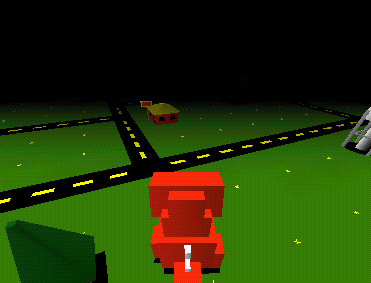One important thing to note about all of the above equations is that each object is dealt with separately. That is, one object does not block light from reaching another object (which is realistic but expensive.)

### Multiple Lights

With multiple lights the affect of all the lights are additive.

We often use polygons to simulate curved surfaces. If these cases we want the colours of the polygons to flow smoothly into each other.

• each entire polygon is drawn with the same colour
• need to know one normal for the entire polygon
• fast
• lighting equation used once per polygon

Given a single normal to the plane the lighting equations and the material properties are used to generate a single colour. The polygon is filled with that colour.

Here is another of the OpenGL samples with a flat shaded scene: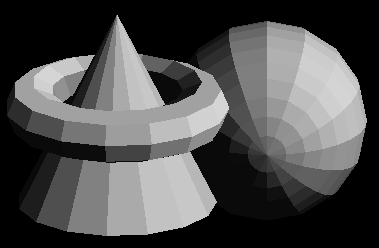• colours are interpolated across the polygon
• need to know a normal for each vertex of the polygon
• lighting equation used at each vertex

Given a normal at each vertex of the polygon, the colour at each vertex is determined from the lighting equations and the material properties. Linear interpolation of the colour values at each vertex are used to generate colour values for each pixel on the edges. Linear interpolation across each scan line is used to then fill in the colour of the polygon.

Here is another of the OpenGL samples with a smooth shaded scene: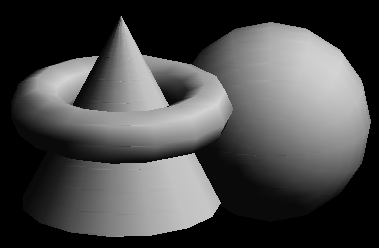• normals are interpolated across the polygon
• need to know a normal for each vertex of the polygon
• better at dealing with highlights than Goraud shading
• lighting equation used at each pixel

Where Goraud shading uses normals at the vertices and then interpolates the resulting colours across the polygon, Phong shading goes further and interpolates tha normals. Linear interpolation of the normal values at each vertex are used to generate normal values for the pixels on the edges. Linear interpolation across each scan line is used to then generate normals at each pixel across the scan line.

Whether we are interpolating normals or colours the procedure is the same: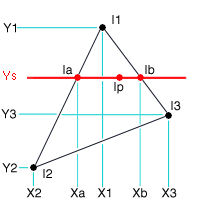To find the intensity of Ip, we need to know the intensity of Ia and Ib. To find the intensity of Ia we need to know the intensity of I1 and I2. To find the intensity of Ib we need to know the intensity of I1 and I3.

Ia = (Ys - Y2) / (Y1 - Y2) * I1 + (Y1 - Ys) / (Y1 - Y2) * I2
Ib = (Ys - Y3) / (Y1 - Y3) * I1 + (Y1 - Ys) / (Y1 - Y3) * I3
Ip = (Xb - Xp) / (Xb - Xa) * Ia + (Xp - Xa) / (Xb - Xa) * Ib

AntiAliasing

Lines and the edges of polygons still look jagged at this point. This is especially noticable when moving through a static scene looking at sharp edges.

This is known as aliasing, and is caused by the conversion from the mathematical edge to a discrete set of pixels. We saw near the beginning of the course how to scan convert a line into the frame buffer, but at that point we only dealth with placing the pixel or not placing the pixel. Now we will deal with coverage.

The mathematical line will likely not exactly cover pixel boundaries - some pixels will be mostly covered by the line (or edge), and others only slightly. Instead of making a yes/no decision we can assign a value to this coverage (from say 0 to 1) for each pixel and then use these values to blend the colour of the line (or edge) with the existing contents of the frame buffer.

In OpenGL you give hints setting the hints for GL_POINT_SMOOTH_HINT, GL_LINE_SMOOTH_HINT, GL_POLYGON_SMOOTH_HINT to tell OpenGL to be GL_FASTEST or GL_NICEST to try and snooth things out using the alpha (transparency) values.

You also need to enable or disable that smoothing

glEnable(GL_POINT_SMOOTH);
glEnable(GL_LINE_SMOOTH);
glEnable(GL_POLYGON_SMOOTH);

### Texture and Bump Mapping

So far we have talked about using polygons and lights to generate the look of all the objects in the scene. When fine detail is needed this may not be the most efficient way.

Texture mapping is the process of taking a 2D image and mapping onto a polygon in the scene. This texture acts like a painting, adding 2D detail to the 2D polygon.

Instead of filling a polygon with a colour in the scan conversion process with fill the pixels of the polygon with the pixels of the texture (texels.)

Used to:

texture space -> object space -> image space

The following images show an increasingly complex sphere texture mapped with the following image of mars.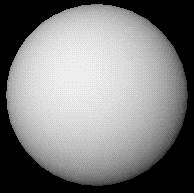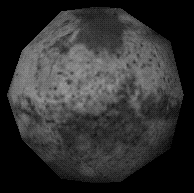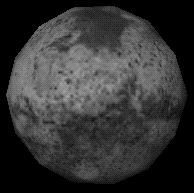Texture maps are flat 2D images. They are often used however to simulate 3D surfaces. Unfortunately the lighting across the polygon tends to make these textures look flat.

Bump mapping is an attempt to get around this problem by using the texture to make the object appear to have more 3D detail than it actually has. A common example of htis would be the small dimples covering a strawberry. Modelling the dimples would be expensive, but just drawing them on the texture doesn't give the correct effect because the texture is flat. With bump mapping the texture affects the surface normals underneath it which in turn modify the lighting at that point.